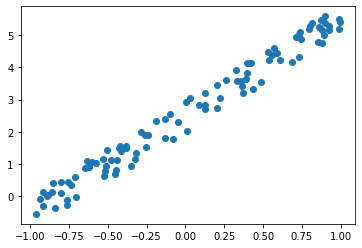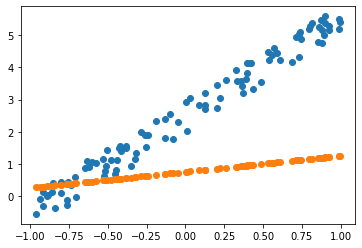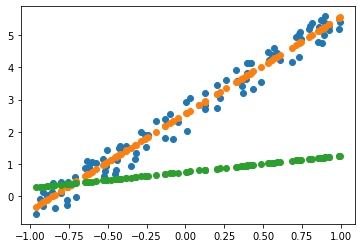## Lesson Video:

This article is also a Jupyter Notebook available to be run from the top down. There will be code snippets that you can then run in any environment.

Below are the versions of fastai, fastcore, and wwf currently running at the time of writing this:

• fastai: 2.1.10
• fastcore: 1.3.13
• wwf: 0.0.5

Grab the related library we will need

from fastai.basics import *


Below you will find the exact imports for everything we use today

import torch
from torch import nn

import numpy as np

import matplotlib.pyplot as plt

from fastai.torch_core import tensor


• Optimization technique (optimizer)
• Commonly used in neural networks
• Example with linear regression

## Linear Regression

• Fit a line on 100 points
n = 100


Generate our data

x = torch.ones(n,2)

len(x), x[:5]

(100, tensor([[1., 1.],
[1., 1.],
[1., 1.],
[1., 1.],
[1., 1.]]))

Randomize it in a uniform distribution from -1 to 1

x[:,0].uniform_(-1., 1)
x[:5], x.shape

(tensor([[-0.7631,  1.0000],
[ 0.8743,  1.0000],
[ 0.3916,  1.0000],
[ 0.8608,  1.0000],
[ 0.2030,  1.0000]]), torch.Size([100, 2]))
• Any linear model is y=mx+b
• m, x, and b are matrices
• We have x
m = tensor(3.,2); m, m.shape

(tensor([3., 2.]), torch.Size())
• b is a random bias
b = torch.rand(n); b[:5], b.shape

(tensor([0.1767, 0.8454, 0.4767, 0.6628, 0.1358]), torch.Size())

Now we can make our y

• Matrix multiplication is denoted with @
y = x@m + b


We'll know if we got a size wrong if:

m@x + b

---------------------------------------------------------------------------
RuntimeError                              Traceback (most recent call last)
<ipython-input-9-ac53957f9814> in <module>()
----> 1 m@x + b

RuntimeError: mat1 and mat2 shapes cannot be multiplied (1x2 and 100x2)

Plot our results

plt.scatter(x[:,0], y)

<matplotlib.collections.PathCollection at 0x7f78991b6be0>Our weights from last lesson should minimize the distance between points and our line.

• mean squared error: Take distance from pred and y, square, then average
def mse(y_hat, y): return ((y_hat-y)**2).mean()


When we run our model, we are trying to predict m

For example, say a = (0.5, 0.75).

• Make a prediction
• Calculate the error
a = tensor(.5, .75)


Make prediction

y_pred = x@a


Calculate error

mse(y_pred, y)

tensor(5.8721)

What does that mean? Let's plot it

plt.scatter(x[:,0],y)
plt.scatter(x[:,0],y_pred)

<matplotlib.collections.PathCollection at 0x7f7899162cc0>Model doesn't seen to quite fit. What's next? Optimization

• Goal: Minimize the loss function (mse)
• Starts with parameters
• Moves towards new parameters to minimize the function
• Take steps in the negative direction of gradient function

First let's make this parameter

a = nn.Parameter(a); a

Parameter containing:
tensor([0.5000, 0.7500], requires_grad=True)

Next let's create an update function to check if the current a improved. If so, move even closer.

We'll print out every 10 iterations to see how we are doing

def update():
y_hat = x@a
loss = mse(y, y_hat)
if i % 10 == 0: print(loss)
loss.backward()

• torch.no_grad: No back propogation (no updating of our weights)
• sub_: Subtracts some value (lr * our gradient)
• grad.zero_: Zeros our gradients
lr = 1e-1

for i in range(100): update()

tensor(5.8721, grad_fn=<MeanBackward0>)


Now let's see how this new a compares.

plt.scatter(x[:,0],y)
plt.scatter(x[:,0], (x@a).detach())
plt.scatter(x[:,0],y_pred)

<matplotlib.collections.PathCollection at 0x7f7898c8aeb8>We fit our line much better here

## Animate the process

from matplotlib import animation, rc
rc('animation', html='jshtml')


Let's redo the process and animate our y closing in

a = nn.Parameter(tensor(0.5, 0.75)); a

Parameter containing:
tensor([0.5000, 0.7500], requires_grad=True)

We'll want to set a new y to our x@a

def animate(i):
update()
line.set_ydata((x@a).detach())
return line,


Let's create a base figure

fig = plt.figure()
plt.scatter(x[:,0], y, c='orange')
line, = plt.plot(x[:,0], (x@a).detach())
plt.close()


And animate!

animation.FuncAnimation(fig, animate, np.arange(0,100), interval=20)